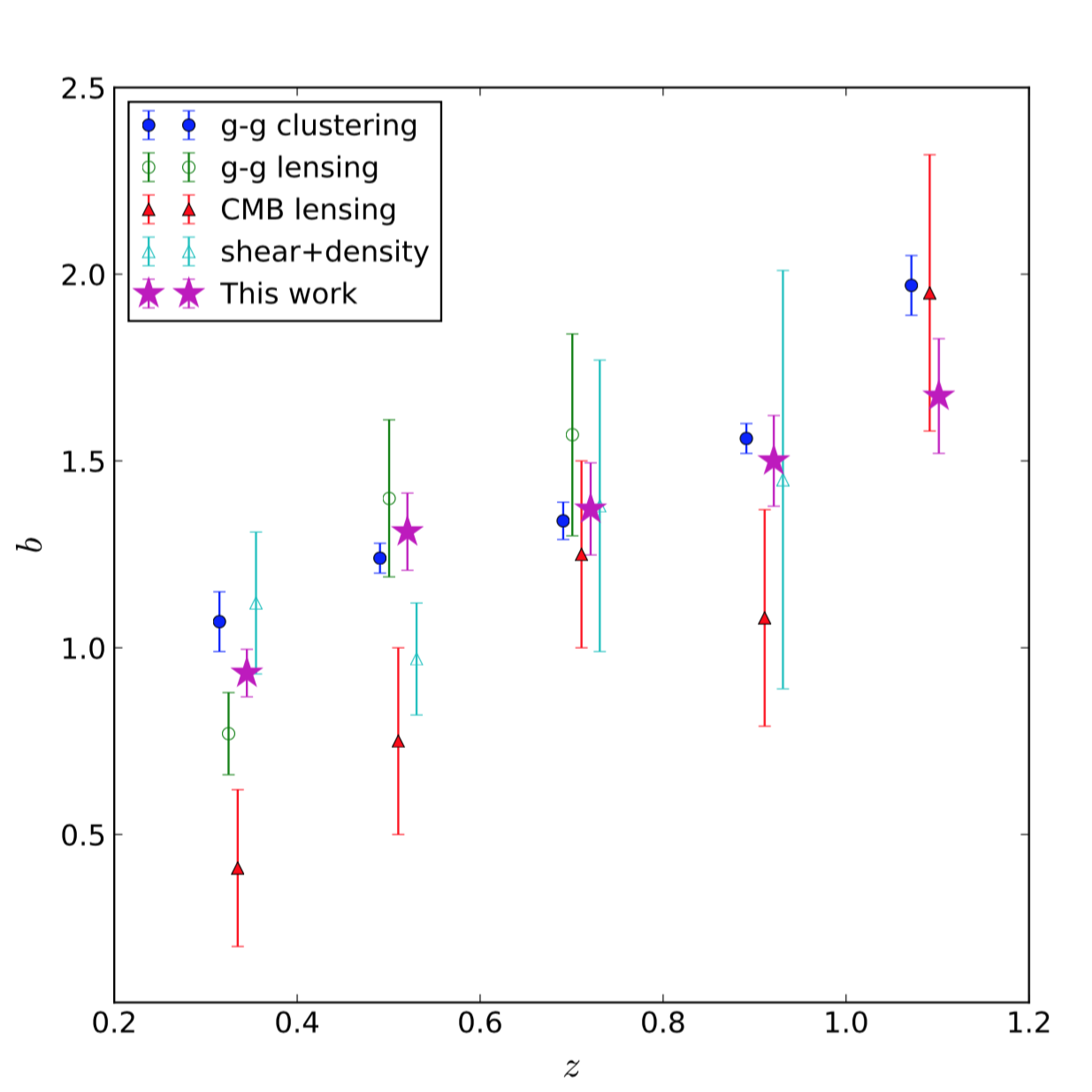# Measuring Linear and Non-linear Galaxy Bias Using Counts-in-Cells in the Dark Energy Survey Science Verification DataAuthors: A. I. Salvador, F. J. Sánchez, A. Pagul et al. Journal: Year: 07/2018 Download: ADS| Arxiv

## Abstract

Non-linear bias measurements require a great level of control of potential systematic effects in galaxy redshift surveys. Our goal is to demonstrate the viability of using Counts-in-Cells (CiC), a statistical measure of the galaxy distribution, as a competitive method to determine linear and higher-order galaxy bias and assess clustering systematics. We measure the galaxy bias by comparing the first four moments of the galaxy density distribution with those of the dark matter distribution. We use data from the MICE simulation to evaluate the performance of this method, and subsequently perform measurements on the public Science Verification (SV) data from the Dark Energy Survey (DES). We find that the linear bias obtained with CiC is consistent with measurements of the bias performed using galaxy-galaxy clustering, galaxy-galaxy lensing, CMB lensing, and shear+clustering measurements. Furthermore, we compute the projected (2D) non-linear bias using the expansion $\delta_{g} = \sum_{k=0}^{3} (b_{k}/k!) \delta^{k}$, finding a non-zero value for $b_2$ at the $3\sigma$ level. We also check a non-local bias model and show that the linear bias measurements are robust to the addition of new parameters. We compare our 2D results to the 3D prediction and find compatibility in the large scale regime ($>30$ Mpc $h^{-1}$)